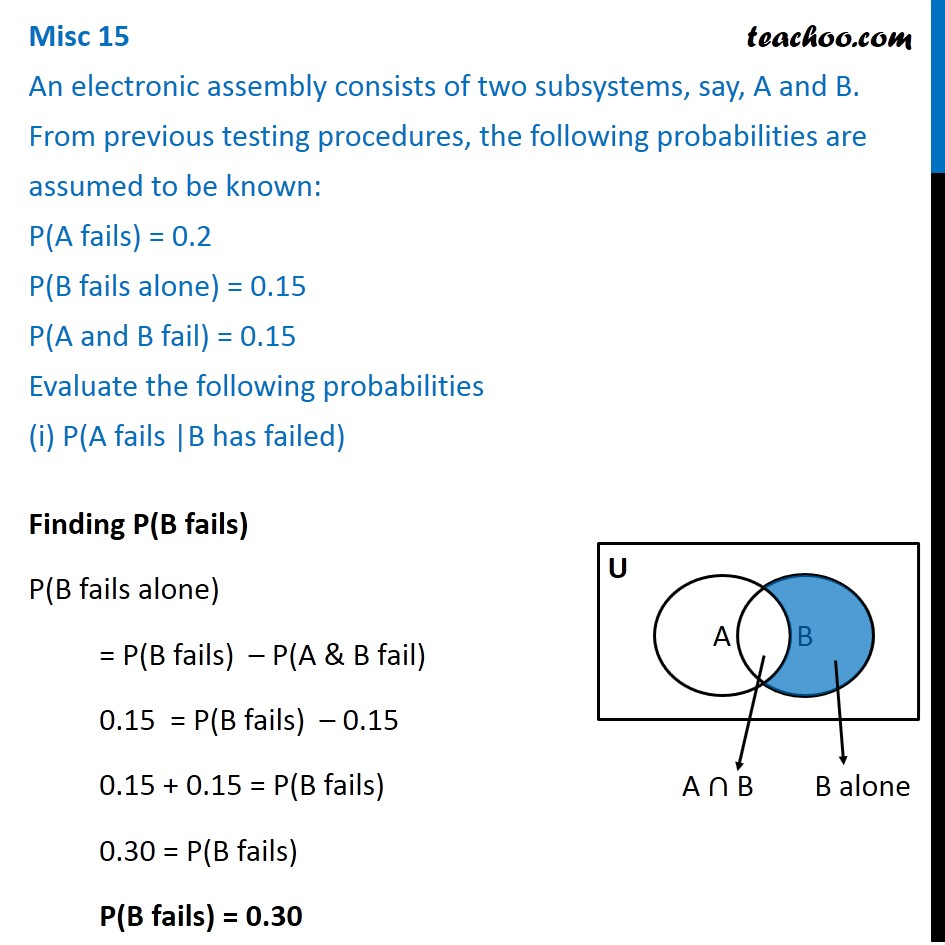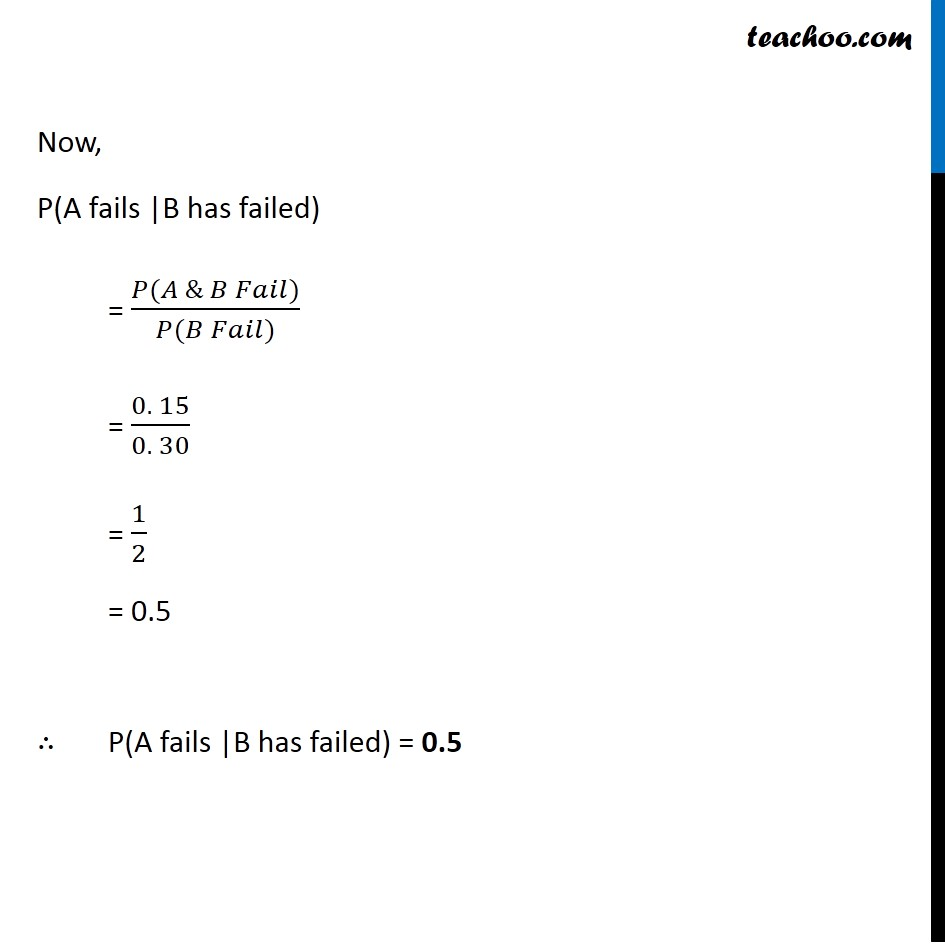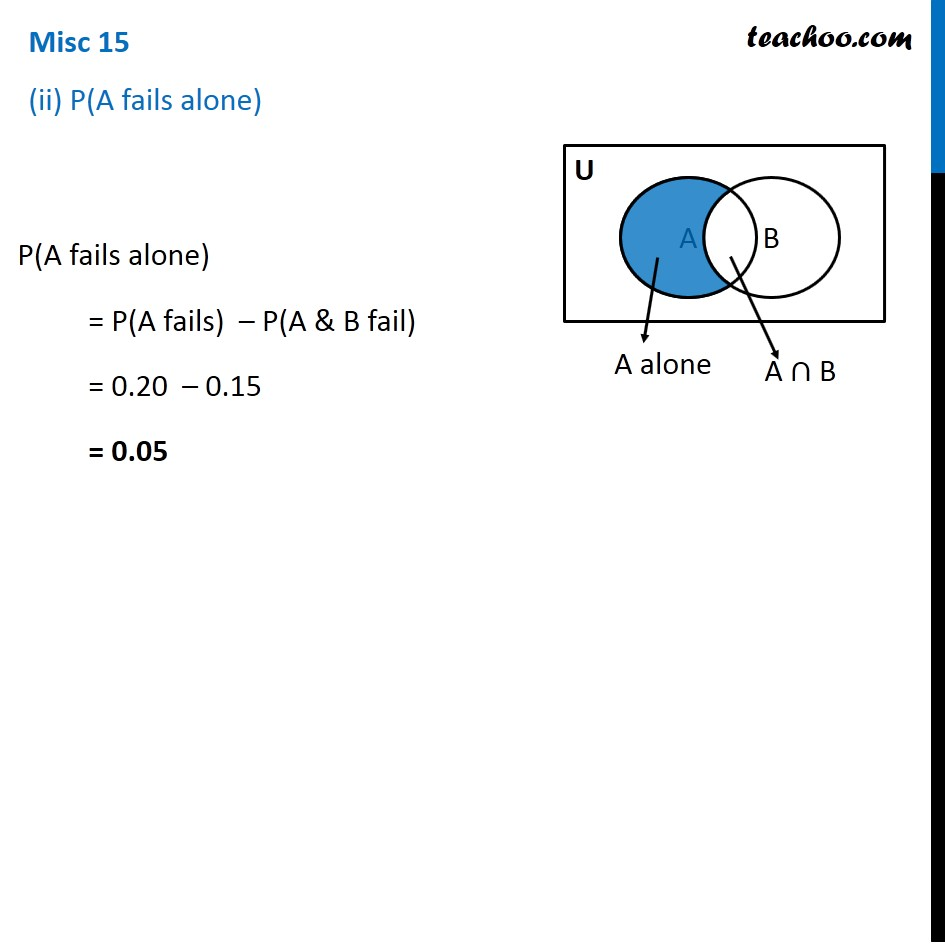Conditional Probability - Values given

Chapter 13 Class 12 Probability
Concept wiseIntroducing your new favourite teacher - Teachoo Black, at only ₹83 per month

### Transcript

Misc 15 An electronic assembly consists of two subsystems, say, A and B. From previous testing procedures, the following probabilities are assumed to be known: P(A fails) = 0.2 P(B fails alone) = 0.15 P(A and B fail) = 0.15 Evaluate the following probabilities (i) P(A fails |B has failed) Now, P(A fails |B has failed) = (𝑃(𝐴 & 𝐵 𝐹𝑎𝑖𝑙))/(𝑃(𝐵 𝐹𝑎𝑖𝑙)) = (0. 15)/(0. 30) = 1/2 = 0.5 ∴ P(A fails |B has failed) = 0.5 Misc 15 (ii) P(A fails alone) P(A fails alone) = P(A fails) – P(A & B fail) = 0.20 – 0.15 = 0.05